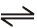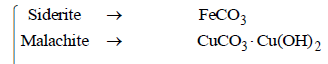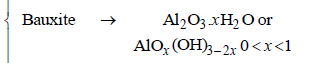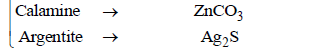Courses

# Matrix-Match Type Questions: General Principles & Processes of Isolation of Elements | JEE Advanced Notes | EduRev

## JEE : Matrix-Match Type Questions: General Principles & Processes of Isolation of Elements | JEE Advanced Notes | EduRev

The document Matrix-Match Type Questions: General Principles & Processes of Isolation of Elements | JEE Advanced Notes | EduRev is a part of the JEE Course Class 12 Chemistry 35 Years JEE Mains &Advance Past yr Paper.
All you need of JEE at this link: JEE

Direction : question contains statements given in two columns, which have to be matched. The statements in Column-I are labelled A, B, C and D, while the statements in Column-II are labelled p, q, r, s and t.

Q.

Match the extraction processes listed in Column I with metals listed in Column II :          (2006 - 6M)

Ans: (A)- p, r; (B) - p, r; (C) - q; (D) - s

 Column I Column II (A) Self reduction (p) Lead (B) Carbon reduction (q) Silver (C) Complex formation and displacement by metal (r) Copper (D) Decomposition of iodide (s) Boron

Solution :

NOTE : The oxides and sulphides of less active metals like Hg, Cu & Pb are unstable to heat and hence no reducing agent is required. They undergo self reduction.
(A) 2Cu2S + 3O2 →2Cu2O+ 2SO2 ;
Cu2S + 2Cu2O →6Cu + SO2

(B) 2PbS + 3O2 → 2PbO + 2SO2 ;
PbS + 2PbO → 3Pb + SO2
Hence (A) → (p), (r)
NOTE : The oxides of less electropositive metals like Pb, Zn, Fe, Sn, Cu, etc. are reduced by strongly heating them with coke or coal.

PbO + C → Pb + CO2 ;
2Cu2O+ C → 4Cu + CO2
Hence (B) → (p), (r)
Extraction from argentite (Ag2S)
Ag2S + 2NaCNNa2S + 2AgCN;
Sod. argentocyanide soluble
AgCN+ NaCN → Na[Ag(CN)2 ]
Zn, being more electropositive than Ag, displaces Ag from the complex.
2Na[Ag(CN)2 ] + Zn → Na2[Zn(CN)4 ] + 2Ag¯
Hence (C) →(q)

Among the halides of boron, BI3 is unstable because of the large size of Iodine and small size of boron atom. Hence it decomposes to give boron. Thus, (D) → (s).

2. Match the conversions in Column I with the type(s) of reaction(s) given in Column II.      (2008 - 6M)

 Column I Column II (A) PbS → PbO (p) roasting (B) CaCO3 → CaO (q) calcination (C) ZnS → Zn (r) carbon reduction (D) Cu2S → Cu (s) self reduction

Ans : (A) - p; (B) - q; (C) - p, r; (D) - p, s

3. Match the anionic species given in Column-I that are present in the ore(s) given in Column-II. (JEE Adv. 2015)

 Column I Column II (A) Carbonate (p) Siderite (B) Sulphide (q) Malachite (C) Hydroxide (r) Bauxite (D) Oxide (s) Calamine (t) Argentite

Ans: (A)- p,q,s, (B)- t, (C)- q, r, (D)- r

Solution :Offer running on EduRev: Apply code STAYHOME200 to get INR 200 off on our premium plan EduRev Infinity!

68 docs|36 tests

,

,

,

,

,

,

,

,

,

,

,

,

,

,

,

,

,

,

,

,

,

;